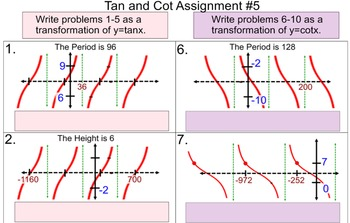# Tangent & Cotangent Transformations, Instruction + 6 Assignments Power PointSubject
Grade Levels
Resource Type
Product Rating
File Type

Compressed Zip File

Be sure that you have an application to open this file type before downloading and/or purchasing.

11 MB
Share
Product Description
These activities are done in degrees.

There are 7 Power Point files in this zip folder. 2 are instruction files and 5 are assignment files.

Power Point Instruction file #1 is a 14 slide Power Point Presentation. The emphasis is defining inflection points as concavity change and sharp changes in direction. These 4 key concepts are introduced for tangent and cotangent graphs:
1. Inflection Points on the centerline
2. Inflection points above the centerline
3. Inflection points below the centerline
4. Asymptotes

Power Point Instruction file #2 is a 28 slide Power Point Presentation. It relates y=tanx to the following:
1. y=-2+tanx
2. y=2tanx
3. y=tan2x
4. y=tan(x-20)
Computer models of y=tanx and the above transformations are used to illustrate the transformations.

.

Power Point Assignment #1 gives 6 graphs (3 tan and 3 cot) where the student has to identify the following for each graph:
1. The vertical shift
2. The vertical stretch
3. The period
4. The horizontal stretch factor
5. The phase angle(3 for tan and 3 for cot)
It is on 1 page for easy printing.

Assignment #2 and #3 are in the same Power Point file.

Assignment #2 is 10 Tan graphs. The student must use transformations to write an equation of each.It is on 1 page for easy printing. Assignment #3 is 10 Cot graphs. The student must use transformations to write an equation of each. It is on 1 page for easy printing.

Power Point Assignment #4 gives 6 graphs (3 tan and 3 cot) where the student has to identify the following for each graph:
1. The vertical shift
2. The vertical stretch
3. The period
4. The horizontal stretch factor
5. The phase angle(3 for tan and 3 for cot)

Power Pointnt Assignment file #4A is like #4. The student has to also find the phase angle of -cot and -tan. It is on 1 page for easy printing.

Power Point Assignment file #5 provides 5 tangent graphs and 5 cotangent graphs. The student is required to write an equation for each graph. It is on 1 page for easy printing.

Solutions are included.

Thank you for looking
Total Pages
N/A
Answer Key
Included
Teaching Duration
4 days
Report this Resource
Reported resources will be reviewed by our team. Report this resource to let us know if this resource violates TpT’s content guidelines.Sign Up